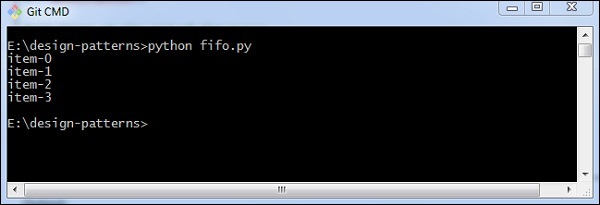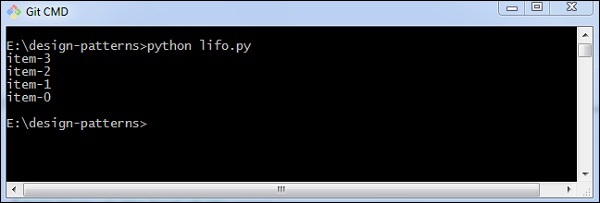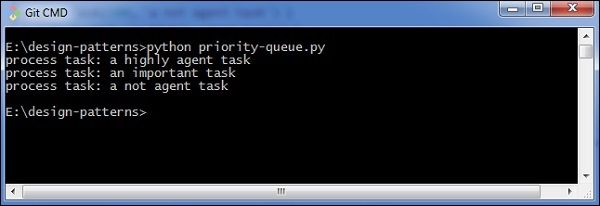# Python Design Patterns - Queues

Queue is a collection of objects, which define a simple data structure following the FIFO (Fast In Fast Out) and the LIFO (Last In First Out) procedures. The insert and delete operations are referred as enqueue and dequeue operations.

## How to implement the FIFO procedure?

The following program helps in the implementation of FIFO −

```import Queue

q = Queue.Queue()

#put items at the end of the queue
for x in range(4):
q.put("item-" + str(x))

#remove items from the head of the queue
while not q.empty():
print q.get()
```

### Output

The above program generates the following output −## How to implement the LIFO procedure?

The following program helps in the implementation of the LIFO procedure −

```import Queue

q = Queue.LifoQueue()

for x in range(4):
q.put("item-" + str(x))

#remove items from the head of the queue
while not q.empty():
print q.get()
```

### Output

The above program generates the following output −## What is a Priority Queue?

Priority queue is a container data structure that manages a set of records with the ordered keys to provide quick access to the record with smallest or largest key in specified data structure.

### How to implement a priority queue?

The implementation of priority queue is as follows −

```import Queue

def __init__(self, priority, name):
self.priority = priority
self.name = name

def __cmp__(self, other):
return cmp(self.priority, other.priority)

q = Queue.PriorityQueue()